Science Journal of Applied Mathematics and Statistics
Volume 3, Issue 4, August 2015, Pages: 184-187

Detection of Non-Linearity in the Time Series Using BDS Test

Akintunde, M. O.*, Oyekunle, J. O., Olalude G. A.

Department of Statistics, School of applied Sciences, Federal Polytechnic, Ede, Osun State, Nigeria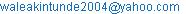(Akintunde
M. O.)

Akintunde, M. O., Oyekunle, J. O., Olalude G. A.. Detection of Non-Linearity in the Time Series Using BDS Test. Science Journal of Applied Mathematics and Statistics. Vol. 3, No. 4, 2015, pp. 184-187. doi: 10.11648/j.sjams.20150304.13

Abstract: The need to determine the status of the series is a very important issue that must be addressed before embarking on the statistical analysis of such series; this paper therefore, examines the status of the commercial bank savings in Nigeria. From the analysis we discovered that at level the series was not stationary as shown in figure1, however at the first difference (figure2) the series was stationary, so also the unit root test applied shows that at level the series was not stationary (table1) but at first difference it was stationary (table2) and this actually paved way for the application of Brock- Dechert-Scheinkman (table3) test which actually revealed that this series could be best estimated by the use of non-linear model as the null hypothesis of linearity of the series was out rightly rejected and the alternative was accepted. The importance of this result lies on the fact that it guides against model misspecification as using linear model to estimate the parameter of the non-linear model will result in model judgmental error.

Keywords: Stationary, Unit Root, BDS Test, Linear Model, Non-Linear Model, Bank Savings

Contents

1. Introduction

Commercial banking in Nigeria has witnessed an era of impressive profitability, characterised by high competition, huge deposits and varied investment opportunities, in an effort to make quick profits, the commercial banks relied essentially on self liquidating loans and diversified their portfolio into less risky investments with safe margin. They are the most important savings, mobilization and financial resource allocation institutions. Consequently, these roles make them an important phenomenon in economic growth and development, in performing these roles; banks need to have the potential, scope and prospects for mobilising financial resources and allocating them to productive investments. Therefore, no matter the sources of income or the economic policies of the country, commercial banks would be interest in giving out loans and advances to their numerous customers bearing in mind the three principles guiding their operations which are profitability, liquidity and solvency.

Savings may be defined as that part of income not immediately spent or consumed but set aside for either immediate or for future consumption, investment or for speculative motive, this is seen as a weapon for economic growth and development. Many factors have been identified in literature to influence savings; and one of these major factors is population and demographic features. (Mohsin et al, 2006;Loayza et al, 2000 and 1999; and Higgins, 1998). The current trend in Nigeria banking and finance sector, suggest that the days of cheap profits are now over and only banks with well conceptualised lending and credit administration policies and procedures can survive the emerging competition. The best evidence that a bank is viable is if it is able to sustain consistent growth of high-quality earnings. It does not follow, however, that the bank will continue to be viable in a changing, regulatory, economic, or competitive environment. Barltrop (1992) opined that it is desirable for a bank to maintain earnings growth at a pace that yields a level of dividends satisfactorily to shareholders, while also reinforcing its capital so that it can maintain an acceptable capital to asset ratio. Abe (1985) posited that deposits are the major source of funding for every licensed bank. A bank depends on a hand-core funding base generated from current, savings and term deposit accounts. To a large extent, the deposit base forms the bedrock of bank’s loan and advances from which it derives its income. Nwadibia (1992) asserts that fixed assets represent the land and building and other fixed assets which are owned by the bank. Akintunde et.al. (2013), opined that a miss-specified model will lead to judgmental error and as such should be avoided as much as possible because of its implication on economic.

2. The Bds Test

There are so many ways of establishing the status of a given series among them are Hinich portmanteau test (1986), C  brook test (1986), H and C- test (1986), BDS test and so many other ways. The attention of this paper is on Brock- Dechert-Scheinkman test (hereafter denoted as the BDS test) was first devised by W.A. Brock, W. Dechert and J. Scheinkman (Brock, Dechert & Scheinkman, 1996) and can be used as a general test of model misspecification. Since the BDS test has reasonable power against the GARCH models, it has been utilized as a diagnostic tool to determine the adequacy of GARCH models for detecting non-linear of the series (Guglielmo M.C. 2005). In this case, the standardized residuals from the fitted GARCH models are subjected to the BDS test under the null hypothesis of sufficient linear components of the series. If the BDS test rejects the null hypothesis using appropriate critical values derived from simulation, then the fitted GARCH model is assumed to be an adequate characterization of the data. The BDS test statistic is calculated as follows. First, the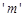- histories’ of the data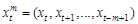are calculated for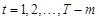for some integer embedding dimension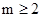. To converts the series of scalars into a series of vectors of overlapping series. Select m value in such a way that the embed time series into m-dimensional vectors, by taking each m successive points in the series.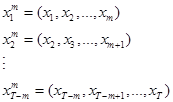The correlation integral is then computed, which counts the proportion of points in m-dimensional hyperspace that are within a distance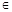of each other.Where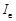is an indicator function that equals one if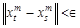and zero otherwise, and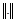denotes the sup. norm. BDS show that under the null hypothesis that the observed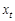are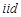, then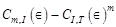with probability one as the sample size tends to infinity andtends to zero. The BDS test statistic, which has a limiting standard normal distribution, then, follows as: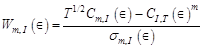where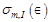and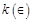is estimated by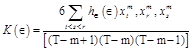and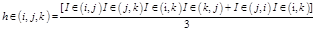Two parameters are to be chosen by the user: the value of(the radius of the hyper-sphere which determines whether two points are ‘close’ or not), and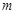(the value of the embedding dimension). Brock et al. (1991) recommend thatis set to between half and three halves the standard deviation of the actual data andis set in line with the number of observations available (for example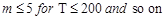) In this study, all combinations of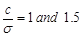(where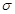is the standard deviation of the sample data), and=2 and 5 are used.

BDS test is a two-tailed test, we should reject the null hypothesis if the BDS test statistic is greater than or less than the critical values (e.g. if a=0.05, the critical value = ±1.96).

A level of significance (a) 5% are usually taken in the hypothesis testing. The series (savings) were analyzed using E-view and the hypothesis was set as follows:

H0: The series are linearly dependent

H1: The series are not linearly dependent

3. Data/ Data Analysis

The data for the paper was extracted from annual report of central bank of Nigeria covering the period from 1961 to 2011. The data covers a period of fifty years. The data was analyzed using Econometric view software (E-view).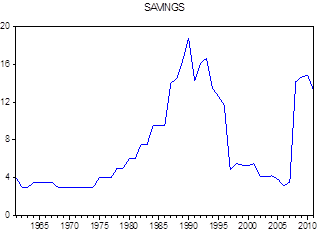Figure 1. Graph of original data.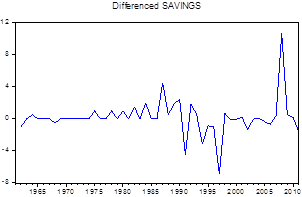Figure 2. Graph of first difference.

The line graph of the series (figures 1) show that the series was not stationary, as a result of the evident of volatility which do not fluctuate around a constant mean. Consequently we looked at the first differences of the series (figures 2) since it has no persistent trend and its values fluctuate around a constant mean of zero.

The stationarity condition of the series can be formally verified by using unit root test (URT) for the leveled and first differences of the series. We test for a unit root using the augmented Dickey-Fuller (ADF) statistic. At level all the series are not stationary but at first difference all series are stationary as shown in tables (1) and (2) below.

Table 1. Series at level.

 Null Hypothesis: SAVINGS has a unit root Exogenous: Constant Lag Length: 0 (Automatic - based on SIC, maxlag=10) t-Statistic Prob.* Augmented Dickey-Fuller test statistic -1.460837 0.5450 Test critical values: 1% level -3.568308 5% level -2.921175 10% level -2.598551 *MacKinnon (1996) one-sided p-values. Augmented Dickey-Fuller Test Equation Dependent Variable: D(SAVINGS) Included observations: 50 after adjustments Variable Coefficient Std. Error t-Statistic Prob. SAVINGS(-1) -0.094021 0.064361 -1.460837 0.1506 C 0.869101 0.561982 1.546494 0.1286 R-squared 0.042567 Mean dependent var 0.184400 Adjusted R-squared 0.022620 S.D. dependent var 2.217706 S.E. of regression 2.192480 Akaike info criterion 4.447122 Sum squared resid 230.7345 Schwarz criterion 4.523603 Log likelihood -109.1780 Hannan-Quinn criter. 4.476246 F-statistic 2.134046 Durbin-Watson stat 1.884090 Prob(F-statistic) 0.150575

Table 2. Series at first difference.

 Null Hypothesis: D(SAVINGS) has a unit root Exogenous: Constant Lag Length: 0 (Automatic based on SIC, MAXLAG=10) t-Statistic Prob.* Augmented Dickey-Fuller test statistic -6.829776 0.0000 Test critical values: 1% level -3.571310 5% level -2.922449 10% level -2.599224 *MacKinnon (1996) one-sided p-values. Augmented Dickey-Fuller Test Equation Dependent Variable: D(SAVINGS,2) Method: Least Squares Included observations: 49 after adjustments Variable Coefficient Std. Error t-Statistic Prob. D(SAVINGS(-1)) -1.000258 0.146455 -6.829776 0.0000 C 0.208629 0.324150 0.643618 0.5230 R-squared 0.498109 Mean dependent var -0.012857 Adjusted R-squared 0.487431 S.D. dependent var 3.153429 S.E. of regression 2.257665 Akaike info criterion 4.506499 Sum squared resid 239.5614 Schwarz criterion 4.583716 Log likelihood -108.4092 F-statistic 46.64584 Durbin-Watson stat 1.987809 Prob(F-statistic) 0.000000

Table 3. BDS Test result for savings

 BDS Test for SAVINGS Included observations: 51 Dimension BDS Statistic Std. Error z-Statistic Prob. 2 0.144246 0.009741 14.80755 0.0000 3 0.221633 0.015731 14.08868 0.0000 4 0.257688 0.019034 13.53808 0.0000 5 0.267745 0.020160 13.28090 0.0000 6 0.291196 0.019759 14.73715 0.0000 Raw epsilon 8.697418 Pairs within epsilon 1847.000 V-Statistic 0.710111 Triples within epsilon 71459.00 V-Statistic 0.538699 Dimension C(m,n) c(m,n) C(1,n-(m-1)) c(1,n-(m-1)) c(1,n-(m-1))^k 2 796.0000 0.649796 871.0000 0.711020 0.505550 3 711.0000 0.604592 854.0000 0.726190 0.382958 4 631.0000 0.559397 836.0000 0.741135 0.301709 5 556.0000 0.514339 817.0000 0.755782 0.246594 6 491.0000 0.474396 780.0000 0.753623 0.183200

This table shows that test statistics is far bigger than the critical values, thus we reject the null hypothesis that the series are linearly dependent. The results actually suggested that the savings in Nigeria economy are non linearly dependent which is a pointer to the chaotic behavior of financial time series data

4. Conclusion

The paper examines the status of the series, it was discovered from the graphs and from the Unit root tests conducted that the series was not stationary at level but stationary at first difference. The BDS test applied reject linearity of the series and from this we concluded that for would be researcher that may be willing to estimate the parameter of this series non-linear model(s) is or are recommended. Anything sort of this will lead to wrong policy formulation.

Recommendations

Examination of the status of the series is a very important aspect in time series and Econometrics research. So researchers should place emphasis on this before embarking on the analysis of the data. This study accordingly recommend for the researchers, policy analyst and academia to always ascertain the status of the series so as not to run into problem(s) of model mis-specification which may eventually lead to wrong judgment.

References

1. Abe, S. I. (1985) Assessment of Bank Performance in Nigeria: Bank examiner view point. Developing a healthy Banking System in Nigeria. Papers and Proceedings of the 1985 Bank Directors’ Seminar FITC, Yaba Lagos
2. Akintunde M. O., Shangodoyin D. K. and Kgosi P. M (2013): Measuring the forecast performance of GARCH and Bilinear-GARCH models in time series data. American Journal of Applied Mathematics
3. Baltrop J. C., (1992) Banking Institutions in Developing Market Interpreting Financial Statements, The World Bank, Washington D.C.
4. Brock, W. A., W. Dechert, & J. Scheinkman. (1996). A test for independence based on the correlation dimension. Working paper, University of Winconsin at Madison, University of Houston, and University of Chicago.
5. Guglielmo M.C. (2005). Generalized autoregressive conditional heteroskedasticity. Journal of Econometrics 31, 307-327
6. Higgins, M. (1998). Demographics, National Savings and International Capital Flows. International Economic Review, No. 39 Pp 343-369.
7. Hsieh, D. A. (1993). Implications of nonlinear dynamics for financial risk management. Journal of Financial and Quantitative Analysis. 28(1): 41 – 64.
8. Kwiatkowski, D., Phillips, P.C.B., Schmidt, P., and Shin, Y. (1992) "Testing the null hypothesis of stationarity against the alternative of a unit root: How sure are we sure that economic time series have a unit root?", Journal of Econometrics, 54, 159-178.
9. Lin, K. (1997) "The ABC’s of BDS." Journal of Computational Intelligence in Finance. 97(July/August): 23 – 26.
10. Loayza N., Schmidt H. K., Serven L., (1999). What Drives Private Savings across the World? Central Bank of Chile Working Paper, No. 47.
11. Loayza, N , K. Schimdt H.K, and Serven L., (2000). What Drives Private Saving across the World? Review of Economics &Statistics, Vol. 82 No. 2 Pp 165-181.
12. Mohsin H., Zeshan A., Muhammad B. (2006). The Impact of Demography, Growth, and Public Policy on Household Saving (A case study of Pakistan). Asia-Pacific Development Journal Vol. 13 No 2.
13. Nwadibia G., (1992). Theory of Money and Banking Freeman Production, Ibadan.

 Contents 1. 2. 3. 4.
Article ToolsAbstractPDF(195K)